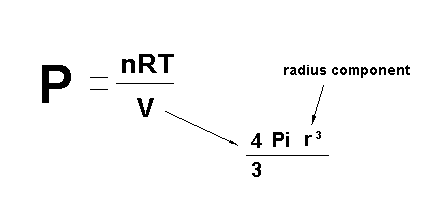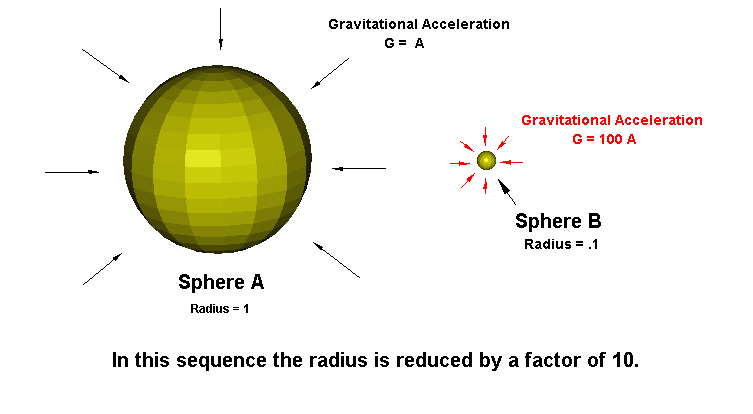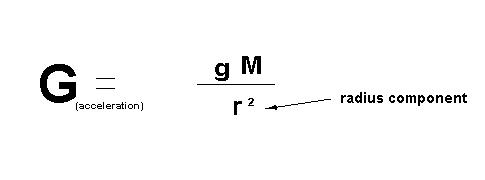The Factor 10 Problem

differentials related to pressure and gravitational subatomic particle flow within a gaseous mass

Original Version for Printing

In this paper, the goal is use Mankind's basic formulas of pressure, a force which compresses a gaseous mass and gravitational subatomic particle flow, again when this force applied to a mass compression occurs. The common factor is volume reduction of matter. Transposing gravity for pressure or the reverse, there is a direct one to one relationship to volume. So how do you equate a factor 10 discrepancy between density and gravity of a sphere where its radius is reduced to .1 of its original size?

This comparison will be defined by a specific set of parameters, the initial cubic area of two spheres will be the same and have a reference mass of the same molecular structure, temperature and density. We will then examine the relationship of pressure vs. gravitational subatomic particle flow and its a affect of a directional force while passing through a mass as the radius of the sphere is reduced by a factor of 10.

Theoretical Concepts of Mankind Affected:

Negative

• Stellar Formation from a Collapsing Nebula Cloud
• Hydrostatic Equilibrium of Stars
• Formation of Planetary Solar Systems

Positive

• The Mass of Stellar and Planetary objects are far greater than currently thought
• The Concept of Gravity as a subatomic particle and the Consideration of the Repulsion ForceFigure 1In this equation mankind's definition of pressure, where the initial mass and its volume is defined in figure 1, the radius component of the sphere for the comparison is reduced by a factor of 10, the initial pressure P within the sphere is increased by a factor of 1,000 as a resultant of the radii reduction. So the P in this equation no matter what its value is a 1000 times greater, yielding 1000P = nRT/V when the radius component is reduced to .1 of original within the spherical V (volume) equation. We can use this formula with our Sun as the matter is in the form or behaves as a gas.  For a simplistic comparison we will let T (temperature) be a average constant for the mass, otherwise the yield would increase in the extremes of stellar cores.

So how does gravitational subatomic particle flow and its affects of compression on a gaseous mass perform under the same set of parameters?Figure 2

 In figure 2 sphere A has the same mass and radii as in figure 1, but we find that gravitational acceleration on the shell of the sphere B when the radii is reduced to .1 of original, gravitational acceleration on the shell of sphere B only increases by a factor of 100. When the factor of T (temperature) is introduced gravity is unaffected, but the outward pressure of the gaseous matter intensifies as a function of molecular motion, thus increasing the differential.The resultant is for every factor 10 reduction of the radii of a gaseous sphere there is a factor 10 increase of pressure within the sphere to the gravitational acceleration upon the shell, which is responsible for containment.

Differential Ratio:

P :: G  with Sphere having radius equal to r

1000P :: 100G with Sphere having radius equal to .1r

10P :: G or a factor 10 deficit

 Stellar Formation from a Collapsing Nebula Cloud Formation of Planetary Solar Systems The current theories on collapsing clouds of dust and gas are invalid due to the factor 10 problem. The concept a cloud self-compressing first due to loss of heat and an increased gravitational flow can not sustain the inward motion of compression towards a centralized point as the increase in pressure along would negate the containment factor of gravity after a few cycles factor 10 reductions of the original radii of the cloud.

 Hydrostatic Equilibrium of Stars When considering current theories on stellar objects we can look to our own Sun as an example. Scientists have concluded that a balance of gravitational acceleration applied to the shell or surface of star or our Sun in this example counters the expansion of the fusion process occurring within the core of the Sun, defined as Hydrostatic Equilibrium. Mankind also assumes the gaseous mass surrounding the core is part of the containment process thus aiding gravity. Would not a hot expanding gas move towards the surface the path of least resistance as opposed to applying pressure to the expanding core? Scientists have not considered all possibilities involved in the theory. What is needed, is to establish a reference radii where gravity is the containment factor at that point, how does it continue to maintain that balanced relationship as the factor 10 problem applies to every uniform 10 reduction of the radius? The factor 10 problem introduces an unbalanced force into the hydrostatic equation of stars, which would lead to a breach of the gravitational containment field.

 The Concept of Gravity and the Consideration of the Repulsion Force Gravity the basis for how the planets of solar system maintain their order, which is based upon the Sun's mass and the equilibrium of planetary orbital velocity vs. orbital distance and various particle flows. Scientists have not considered that the a simplified factor 10 problem negates any balance of gravitational field inflow vs. the pressure output of the fusion process and general molecular compression if a reference radii is established and the hydrostatic equilibrium of the Sun's mass at that point was stable. As the density of the Sun increased, the factor 10 problem would destabilize the hydrostatic equilibrium quickly. In order for the gravitational shell, which contains the outward motion of the Sun, the mass needed for containment would have to be greater than mankind's calculations, thus the balance of planetary orbital velocities and related distances from the Sun could not exist with the increased mass needed to hold together the Sun. Only a massive iron based core in the heart of the Sun would account for not only the gravitational containment force, but also provide the base for its magnetic field. The deciding factor is the introduction of a new force that keeps universal matter apart, the introduction of the repulsion force.

Related Articles:

Magnetic Stars

Universal Laws of Gravity and Repulsion

Planetary Formation

Fusion Process of the Sun